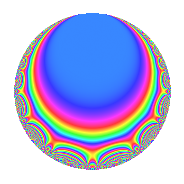# Properties

 Label 6003.2.a.gLevel 6003 Weight 2 Character orbit 6003.a Self dual Yes Analytic conductor 47.934 Analytic rank 0 Dimension 4 CM No Inner twists 1

# Related objects

## Newspace parameters

 Level: $$N$$ = $$6003 = 3^{2} \cdot 23 \cdot 29$$ Weight: $$k$$ = $$2$$ Character orbit: $$[\chi]$$ = 6003.a (trivial)

## Newform invariants

 Self dual: Yes Analytic conductor: $$47.9341963334$$ Analytic rank: $$0$$ Dimension: $$4$$ Coefficient field: 4.4.5744.1 Coefficient ring: $$\Z[a_1, \ldots, a_{17}]$$ Coefficient ring index: $$2$$ Fricke sign: $$-1$$ Sato-Tate group: $\mathrm{SU}(2)$

## $q$-expansion

Coefficients of the $$q$$-expansion are expressed in terms of a basis $$1,\beta_1,\beta_2,\beta_3$$ for the coefficient ring described below. We also show the integral $$q$$-expansion of the trace form.

 $$f(q)$$ $$=$$ $$q$$ $$-2 q^{4}$$ $$+ \beta_{1} q^{5}$$ $$+ ( -1 - \beta_{2} + \beta_{3} ) q^{7}$$ $$+O(q^{10})$$ $$q$$ $$-2 q^{4}$$ $$+ \beta_{1} q^{5}$$ $$+ ( -1 - \beta_{2} + \beta_{3} ) q^{7}$$ $$+ ( 1 - \beta_{1} + \beta_{2} + \beta_{3} ) q^{11}$$ $$+ ( 1 + 2 \beta_{2} ) q^{13}$$ $$+ 4 q^{16}$$ $$+ ( -1 - \beta_{2} + 2 \beta_{3} ) q^{17}$$ $$+ ( 2 + 2 \beta_{2} + \beta_{3} ) q^{19}$$ $$-2 \beta_{1} q^{20}$$ $$- q^{23}$$ $$+ ( -2 + \beta_{1} - \beta_{2} + \beta_{3} ) q^{25}$$ $$+ ( 2 + 2 \beta_{2} - 2 \beta_{3} ) q^{28}$$ $$- q^{29}$$ $$+ ( -1 + 2 \beta_{1} - \beta_{2} + \beta_{3} ) q^{31}$$ $$+ ( -1 + \beta_{1} + \beta_{2} + \beta_{3} ) q^{35}$$ $$+ ( -1 + 3 \beta_{1} - \beta_{2} ) q^{37}$$ $$+ ( -2 + \beta_{1} + 2 \beta_{3} ) q^{41}$$ $$+ ( 1 - 3 \beta_{1} - \beta_{2} ) q^{43}$$ $$+ ( -2 + 2 \beta_{1} - 2 \beta_{2} - 2 \beta_{3} ) q^{44}$$ $$-\beta_{1} q^{47}$$ $$+ ( 2 - \beta_{1} + \beta_{2} - \beta_{3} ) q^{49}$$ $$+ ( -2 - 4 \beta_{2} ) q^{52}$$ $$+ ( 4 - 2 \beta_{1} + 2 \beta_{2} - 2 \beta_{3} ) q^{53}$$ $$+ ( -2 + 2 \beta_{3} ) q^{55}$$ $$+ ( 6 - 2 \beta_{1} - \beta_{2} + \beta_{3} ) q^{59}$$ $$+ ( -4 - 2 \beta_{1} - 2 \beta_{2} ) q^{61}$$ $$-8 q^{64}$$ $$+ ( 2 - \beta_{1} - 2 \beta_{2} + 2 \beta_{3} ) q^{65}$$ $$+ ( 2 + 2 \beta_{2} - 2 \beta_{3} ) q^{67}$$ $$+ ( 2 + 2 \beta_{2} - 4 \beta_{3} ) q^{68}$$ $$+ ( 5 + \beta_{1} - 2 \beta_{3} ) q^{71}$$ $$+ ( -5 + \beta_{2} - \beta_{3} ) q^{73}$$ $$+ ( -4 - 4 \beta_{2} - 2 \beta_{3} ) q^{76}$$ $$+ ( -2 + 4 \beta_{1} - 4 \beta_{2} - 2 \beta_{3} ) q^{77}$$ $$+ ( -6 + 2 \beta_{1} + 3 \beta_{3} ) q^{79}$$ $$+ 4 \beta_{1} q^{80}$$ $$+ ( 6 - 3 \beta_{1} + 4 \beta_{2} ) q^{83}$$ $$+ ( -1 + 2 \beta_{1} + \beta_{2} + 3 \beta_{3} ) q^{85}$$ $$+ ( -1 + 6 \beta_{1} - 3 \beta_{2} ) q^{89}$$ $$+ ( -11 + 6 \beta_{1} - 3 \beta_{2} - \beta_{3} ) q^{91}$$ $$+ 2 q^{92}$$ $$+ ( 2 + \beta_{1} - 2 \beta_{2} + 4 \beta_{3} ) q^{95}$$ $$+ ( -4 + 2 \beta_{1} - 2 \beta_{2} ) q^{97}$$ $$+O(q^{100})$$ $$\operatorname{Tr}(f)(q)$$ $$=$$ $$4q$$ $$\mathstrut -\mathstrut 8q^{4}$$ $$\mathstrut +\mathstrut 2q^{5}$$ $$\mathstrut -\mathstrut 2q^{7}$$ $$\mathstrut +\mathstrut O(q^{10})$$ $$4q$$ $$\mathstrut -\mathstrut 8q^{4}$$ $$\mathstrut +\mathstrut 2q^{5}$$ $$\mathstrut -\mathstrut 2q^{7}$$ $$\mathstrut +\mathstrut 16q^{16}$$ $$\mathstrut -\mathstrut 2q^{17}$$ $$\mathstrut +\mathstrut 4q^{19}$$ $$\mathstrut -\mathstrut 4q^{20}$$ $$\mathstrut -\mathstrut 4q^{23}$$ $$\mathstrut -\mathstrut 4q^{25}$$ $$\mathstrut +\mathstrut 4q^{28}$$ $$\mathstrut -\mathstrut 4q^{29}$$ $$\mathstrut +\mathstrut 2q^{31}$$ $$\mathstrut -\mathstrut 4q^{35}$$ $$\mathstrut +\mathstrut 4q^{37}$$ $$\mathstrut -\mathstrut 6q^{41}$$ $$\mathstrut -\mathstrut 2q^{47}$$ $$\mathstrut +\mathstrut 4q^{49}$$ $$\mathstrut +\mathstrut 8q^{53}$$ $$\mathstrut -\mathstrut 8q^{55}$$ $$\mathstrut +\mathstrut 22q^{59}$$ $$\mathstrut -\mathstrut 16q^{61}$$ $$\mathstrut -\mathstrut 32q^{64}$$ $$\mathstrut +\mathstrut 10q^{65}$$ $$\mathstrut +\mathstrut 4q^{67}$$ $$\mathstrut +\mathstrut 4q^{68}$$ $$\mathstrut +\mathstrut 22q^{71}$$ $$\mathstrut -\mathstrut 22q^{73}$$ $$\mathstrut -\mathstrut 8q^{76}$$ $$\mathstrut +\mathstrut 8q^{77}$$ $$\mathstrut -\mathstrut 20q^{79}$$ $$\mathstrut +\mathstrut 8q^{80}$$ $$\mathstrut +\mathstrut 10q^{83}$$ $$\mathstrut -\mathstrut 2q^{85}$$ $$\mathstrut +\mathstrut 14q^{89}$$ $$\mathstrut -\mathstrut 26q^{91}$$ $$\mathstrut +\mathstrut 8q^{92}$$ $$\mathstrut +\mathstrut 14q^{95}$$ $$\mathstrut -\mathstrut 8q^{97}$$ $$\mathstrut +\mathstrut O(q^{100})$$

Basis of coefficient ring in terms of a root $$\nu$$ of $$x^{4}\mathstrut -\mathstrut$$ $$5$$ $$x^{2}\mathstrut -\mathstrut$$ $$2$$ $$x\mathstrut +\mathstrut$$ $$1$$:

 $$\beta_{0}$$ $$=$$ $$1$$ $$\beta_{1}$$ $$=$$ $$\nu^{3} - 4 \nu - 1$$ $$\beta_{2}$$ $$=$$ $$\nu^{2} - \nu - 3$$ $$\beta_{3}$$ $$=$$ $$-\nu^{3} + \nu^{2} + 5 \nu - 1$$
 $$1$$ $$=$$ $$\beta_0$$ $$\nu$$ $$=$$ $$($$$$\beta_{3}\mathstrut -\mathstrut$$ $$\beta_{2}\mathstrut +\mathstrut$$ $$\beta_{1}\mathstrut -\mathstrut$$ $$1$$$$)/2$$ $$\nu^{2}$$ $$=$$ $$($$$$\beta_{3}\mathstrut +\mathstrut$$ $$\beta_{2}\mathstrut +\mathstrut$$ $$\beta_{1}\mathstrut +\mathstrut$$ $$5$$$$)/2$$ $$\nu^{3}$$ $$=$$ $$2$$ $$\beta_{3}\mathstrut -\mathstrut$$ $$2$$ $$\beta_{2}\mathstrut +\mathstrut$$ $$3$$ $$\beta_{1}\mathstrut -\mathstrut$$ $$1$$

## Embeddings

For each embedding $$\iota_m$$ of the coefficient field, the values $$\iota_m(a_n)$$ are shown below.

For more information on an embedded modular form you can click on its label.

Label $$\iota_m(\nu)$$ $$a_{2}$$ $$a_{3}$$ $$a_{4}$$ $$a_{5}$$ $$a_{6}$$ $$a_{7}$$ $$a_{8}$$ $$a_{9}$$ $$a_{10}$$
1.1
 0.291367 −1.92022 −0.751024 2.37988
0 0 −2.00000 −2.14073 0 2.72347 0 0 0
1.2 0 0 −2.00000 −0.399447 0 −3.44099 0 0 0
1.3 0 0 −2.00000 1.58049 0 −3.08254 0 0 0
1.4 0 0 −2.00000 2.95969 0 1.80007 0 0 0
 $$n$$: e.g. 2-40 or 990-1000 Significant digits: Format: Complex embeddings Normalized embeddings Satake parameters Satake angles

## Inner twists

This newform does not admit any (nontrivial) inner twists.

## Atkin-Lehner signs

$$p$$ Sign
$$3$$ $$-1$$
$$23$$ $$1$$
$$29$$ $$1$$

## Hecke kernels

This newform can be constructed as the intersection of the kernels of the following linear operators acting on $$S_{2}^{\mathrm{new}}(\Gamma_0(6003))$$:

 $$T_{2}$$ $$T_{5}^{4}$$ $$\mathstrut -\mathstrut 2 T_{5}^{3}$$ $$\mathstrut -\mathstrut 6 T_{5}^{2}$$ $$\mathstrut +\mathstrut 8 T_{5}$$ $$\mathstrut +\mathstrut 4$$